# 12.6: Region of Convergence for the Z-Transform

•• Richard Baraniuk et al.
• Victor E. Cameron Professor (Electrical and Computer Engineering) at Rice University

## Introduction

With the z-transform, the s-plane represents a set of signals (complex exponentials (Section 1.8)). For any given LTI (Section 2.1) system, some of these signals may cause the output of the system to converge, while others cause the output to diverge ("blow up"). The set of signals that cause the system's output to converge lie in the region of convergence (ROC). This module will discuss how to find this region of convergence for any discrete-time, LTI system.

## The Region of Convergence

The region of convergence, known as the ROC, is important to understand because it defines the region where the z-transform exists. The z-transform of a sequence is defined as

$X(z)=\sum_{n=-\infty}^{\infty} x[n] z^{-n}$

The ROC for a given $$x[n]$$, is defined as the range of $$z$$ for which the z-transform converges. Since the z-transform is a power series, it converges when $$x[n]z^{−n}$$ is absolutely summable. Stated differently,

$\sum_{n=-\infty}^{\infty}\left|x[n] z^{-n}\right|<\infty$

must be satisfied for convergence.

### Properties of the Region of Convergencec

The Region of Convergence has a number of properties that are dependent on the characteristics of the signal, $$x[n]$$.

• The ROC cannot contain any poles. By definition a pole is a where $$X(z)$$ is infinite. Since $$X(z)$$ must be finite for all $$z$$ for convergence, there cannot be a pole in the ROC.
• If $$\bf{x[n]}$$ is a finite-duration sequence, then the ROC is the entire z-plane, except possibly $$\bf{z=0}$$ or $$\bf{|z|=\infty}$$. A finite-duration sequence is a sequence that is nonzero in a finite interval $$n_1≤n≤n_2$$. As long as each value of $$x[n]$$ is finite then the sequence will be absolutely summable. When $$n_2>0$$ there will be a $$z^{−1}$$ term and thus the ROC will not include $$z=0$$. When $$n_1<0$$ then the sum will be infinite and thus the ROC will not include $$|z|=\infty$$. On the other hand, when $$n_2≤0$$ then the ROC will include $$z=0$$, and when $$n_1≥0$$ the ROC will include $$|z|=\infty$$. With these constraints, the only signal, then, whose ROC is the entire z-plane is $$x[n]=c \delta[n]$$.Figure $$\PageIndex{1}$$: An example of a finite duration sequence.

The next properties apply to infinite duration sequences. As noted above, the z-transform converges when $$|X(z)|<\infty$$. So we can write

$|X(z)|=\left|\sum_{n=-\infty}^{\infty} x[n] z^{-n}\right| \leq \sum_{n=-\infty}^{\infty}\left|x[n] z^{-n}\right|=\sum_{n=-\infty}^{\infty}|x[n]|(|z|)^{-n}$

We can then split the infinite sum into positive-time and negative-time portions. So

$|X(z)| \leq N(z)+P(z)$

where

$N(z)=\sum_{n=-\infty}^{-1}|x[n]|(|z|)^{-n}$

and

$P(z)=\sum_{n=0}^{\infty}|x[n]|(|z|)^{-n}$

In order for $$|X(z)|$$ to be finite, $$|x[n]|$$ must be bounded. Let us then set

$|x(n)| \leq C_{1} r_{1}^{n}$

for

$n<0 \nonumber$

and

$|x(n)| \leq C_{2} r_{2}^{n}$

for

$n≥0 \nonumber$

From this some further properties can be derived:

• If $$\bf{x[n]}$$ is a right-sided sequence, then the ROC extends outward from the outermost pole in $$\bf{X(z)}$$. A right-sided sequence is a sequence where $$x[n]=0$$ for $$n<n_1<\infty$$. Looking at the positive-time portion from the above derivation, it follows that

$P(z) \leq C_{2} \sum_{n=0}^{\infty} r_{2}^{n}(|z|)^{-n}=C_{2} \sum_{n=0}^{\infty}\left(\frac{r_{2}}{|z|}\right)^{n}$

Thus in order for this sum to converge, $$|z|>r_2$$, and therefore the ROC of a right-sided sequence is of the form $$|z|>r_2$$.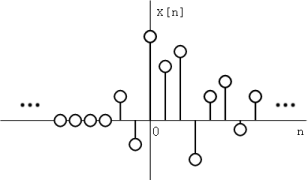Figure $$\PageIndex{2}$$: A right-sided sequence.Figure $$\PageIndex{3}$$: The ROC of a right-sided sequence.
• If $$\bf{x[n]}$$ is a left-sided sequence, then the ROC extends inward from the innermost pole in $$\bf{X(z)}$$. A left-sided sequence is a sequence where $$x[n]=0$$ for $$n>n_2>−\infty$$. Looking at the negative-time portion from the above derivation, it follows that

$N(z) \leq C_{1} \sum_{n=-\infty}^{-1} r_{1}^{n}(|z|)^{-n}=C_{1} \sum_{n=-\infty}^{-1}\left(\frac{r_{1}}{|z|}\right)^{n}=C_{1} \sum_{k=1}^{\infty}\left(\frac{|z|}{r_{1}}\right)^{k}$

Thus in order for this sum to converge, $$|z|<r_1$$, and therefore the ROC of a left-sided sequence is of the form $$|z|<r_1$$.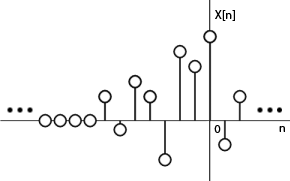Figure $$\PageIndex{4}$$: A left-sided sequence.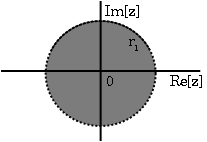Figure $$\PageIndex{5}$$: The ROC of a left-sided sequence.
• If $$\bf{x[n]}$$ is a two-sided sequence, the ROC will be a ring in the z-plane that is bounded on the interior and exterior by a pole. A two-sided sequence is an sequence with infinite duration in the positive and negative directions. From the derivation of the above two properties, it follows that if $$-r_2<|z|<r_2$$ converges, then both the positive-time and negative-time portions converge and thus $$X(z)$$ converges as well. Therefore the ROC of a two-sided sequence is of the form $$-r_2<|z|<r_2$$.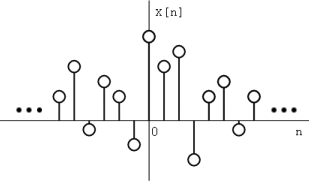Figure $$\PageIndex{6}$$: A two-sided sequence.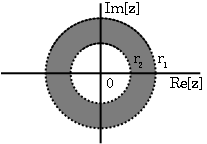Figure $$\PageIndex{7}$$: The ROC of a two-sided sequence.

### Examples

Example $$\PageIndex{1}$$

Let's take

$x_{1}[n]=\left(\frac{1}{2}\right)^{n} u[n]+\left(\frac{1}{4}\right)^{n} u[n]$

The z-transform of $$\left(\frac{1}{2}\right)^{n} u[n]$$ is $$\frac{z}{z-\frac{1}{2}}$$ with an ROC at $$|z|>\frac{1}{2}$$.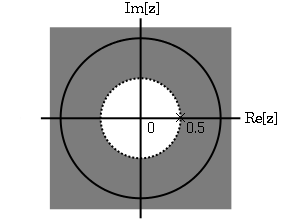Figure $$\PageIndex{8}$$: The ROC of $$\left(\frac{1}{2}\right)^{n} u[n]$$

The z-transform of $$\left(\frac{-1}{4}\right)^{n} u[n]$$ is $$\frac{z}{z+\frac{1}{4}}$$ with an ROC at $$|z|>\frac{-1}{4}$$.Figure $$\PageIndex{9}$$: The ROC of $$\left(\frac{-1}{4}\right)^{n} u[n]$$

Due to linearity,

\begin{align} X_{1}[z] &=\frac{z}{z-\frac{1}{2}}+\frac{z}{z+\frac{1}{4}} \nonumber \\ &=\frac{2 z\left(z-\frac{1}{8}\right)}{\left(z-\frac{1}{2}\right)\left(z+\frac{1}{4}\right)} \end{align}

By observation it is clear that there are two zeros, at $$0$$ and $$\frac{1}{8}$$, and two poles, at $$\frac{1}{2}$$, and $$\frac{−1}{4}$$. Following the obove properties, the ROC is $$|z|>\frac{1}{2}$$.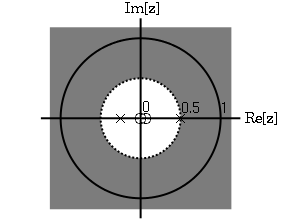Figure $$\PageIndex{10}$$: The ROC of $$x_{1}[n]=\left(\frac{1}{2}\right)^{n} u[n]+\left(\frac{-1}{4}\right)^{n} u[n]$$

Example $$\PageIndex{2}$$

Now take

$x_{2}[n]=\left(\frac{-1}{4}\right)^{n} u[n]-\left(\frac{1}{2}\right)^{n} u[(-n)-1]$

The z-transform and ROC of $$\left(\frac{-1}{4}\right)^{n} u[n]$$ was shown in the example above. The z-transorm of $$\left(-\left(\frac{1}{2}\right)^{n}\right) u[(-n)-1]$$ is $$\frac{z}{z-\frac{1}{2}}$$ with an ROC at $$|z|>\frac{1}{2}$$.Figure $$\PageIndex{11}$$: The ROC of $$\left(-\left(\frac{1}{2}\right)^{n}\right) u[(-n)-1]$$

Once again, by linearity,

\begin{align} X_{2}[z] &=\frac{z}{z+\frac{1}{4}}+\frac{z}{z-\frac{1}{2}} \nonumber \\ &=\frac{z\left(2 z-\frac{1}{8}\right)}{\left(z+\frac{1}{4}\right)\left(z-\frac{1}{2}\right)} \end{align}

By observation it is again clear that there are two zeros, at $$0$$ and $$\frac{1}{16}$$, and two poles, at $$\frac{1}{2}$$, and $$\frac{−1}{4}$$. in ths case though, the ROC is $$|z|<\frac{1}{2}$$.Figure $$\PageIndex{12}$$: The ROC of $$x_{2}[n]=\left(\frac{-1}{4}\right)^{n} u[n]-\left(\frac{1}{2}\right)^{n} u[(-n)-1]$$.

## Graphical Understanding of ROC

Using the demonstration, learn about the region of convergence for the Laplace Transform.

## Conclusion

Clearly, in order to craft a system that is actually useful by virtue of being causal and BIBO stable, we must ensure that it is within the Region of Convergence, which can be ascertained by looking at the pole zero plot. The Region of Convergence is the area in the pole/zero plot of the transfer function in which the function exists. For purposes of useful filter design, we prefer to work with rational functions, which can be described by two polynomials, one each for determining the poles and the zeros, respectively.